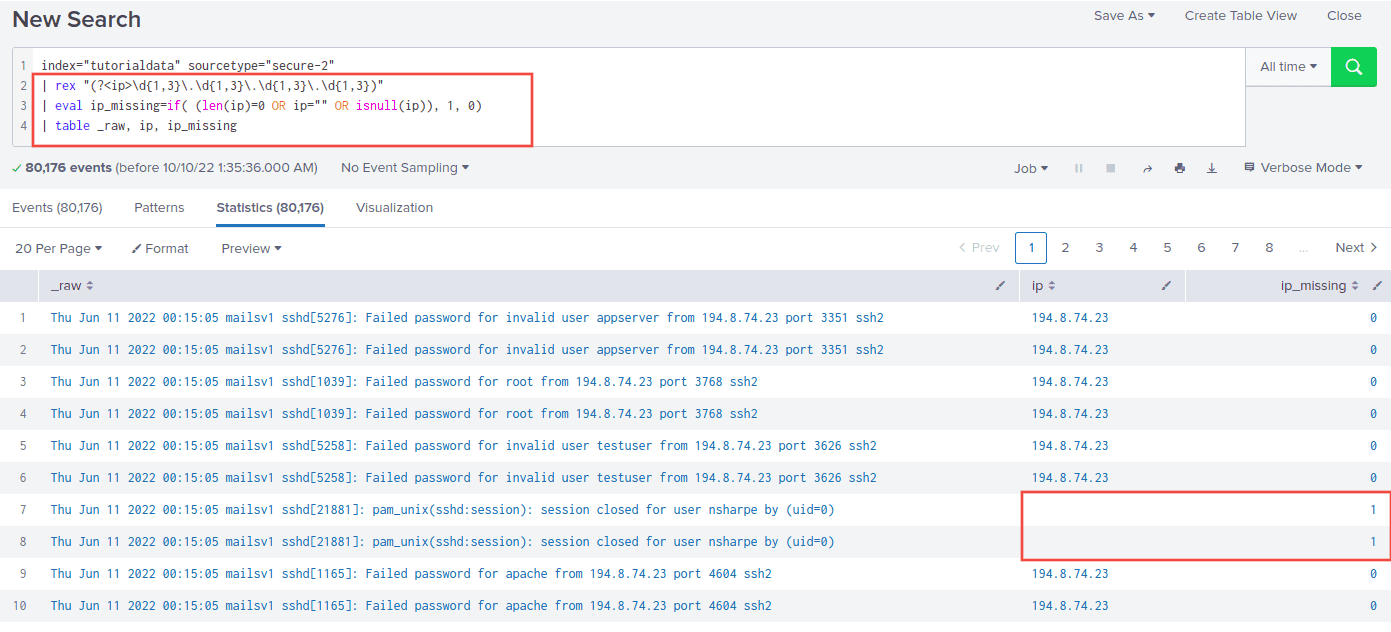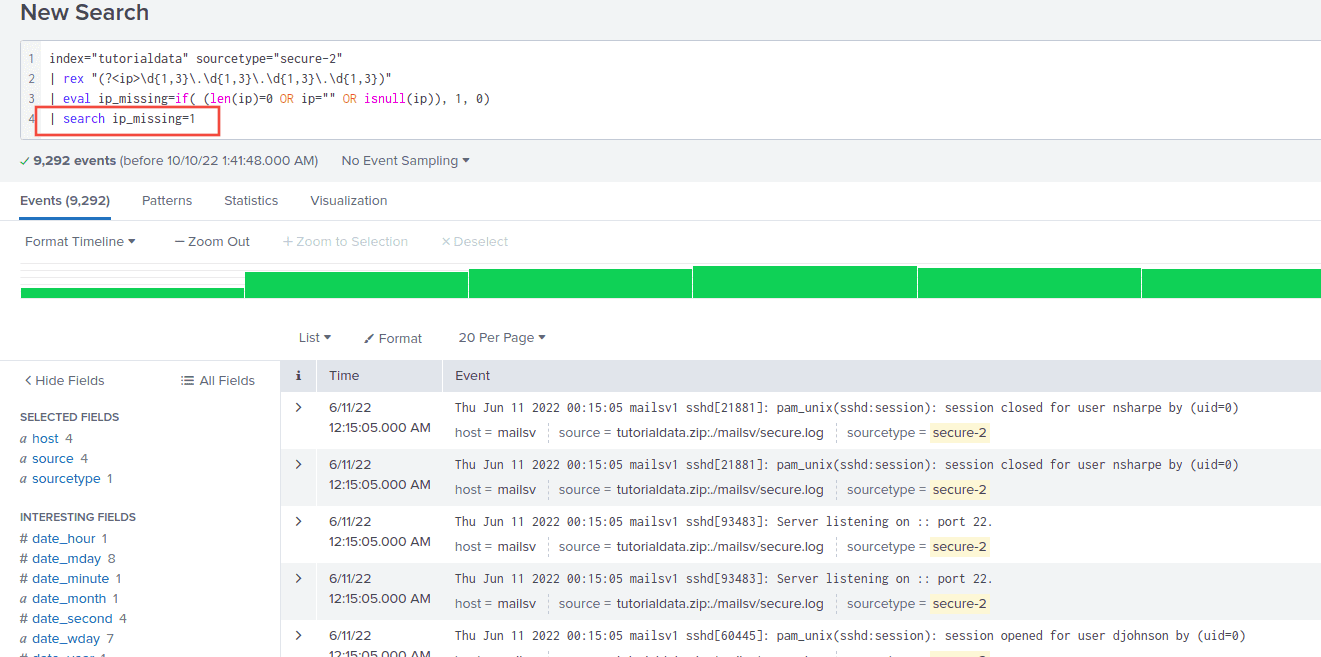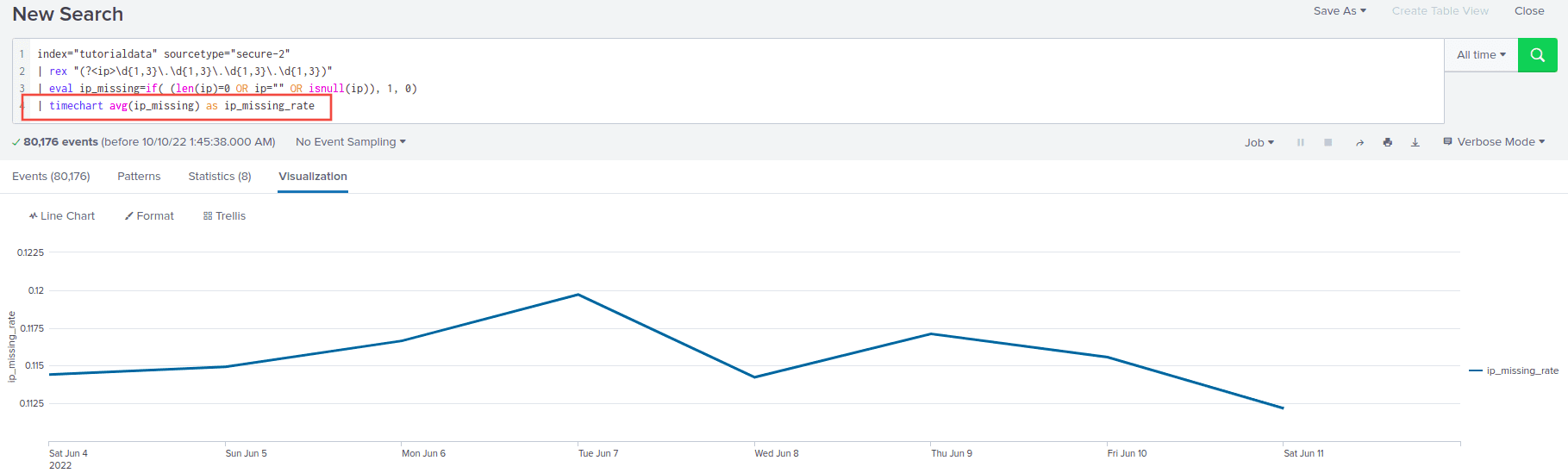Splunk examples: Dealing with null/empty values

# Splunk examples: Dealing with null/empty values

Last updated:

Splunk version used: 8.x. Examples use the tutorial data from Splunk

## Field is null

There are easier ways to do this (using regex), this is just for teaching purposes

It's a bit confusing but this is one of the most robust patterns to filter NULL-ish values in splunk, using a combination of eval and if:

| eval field_missing=if( (len(fieldname)=0 OR fieldname="" OR isnull(fieldname)), 1, 0)


Example: try to extract an IP from the body and flag the rows where it's missing or empty

index="tutorialdata" sourcetype="secure-2"
| rex "(?<ip>\d{1,3}\.\d{1,3}\.\d{1,3}\.\d{1,3})"
| eval ip_missing=if( (len(ip)=0 OR ip="" OR isnull(ip)), 1, 0)In cases where there is no IP in the body, field ip_missing
will be 1, then you can filter by that (see below)

## Where field is null

Same as above, with an extra search at the end:

| eval fieldname_missing=if( (len(fieldname)=0 OR fieldname="" OR isnull(fieldname)), 1, 0)
| search fieldname_missing=1


Example: Select rows where ip is null, empty or missing:

index="tutorialdata" sourcetype="secure-2"
| rex "(?<ip>\d{1,3}\.\d{1,3}\.\d{1,3}\.\d{1,3})"
| eval ip_missing=if( (len(ip)=0 OR ip="" OR isnull(ip)), 1, 0)
| search ip_missing=1Only rows where there are no IPs in the body

## Rate of missing values

A bit similar to the above, just plot the avg of the field

Example: Plot the missing rate for ip per unit time

index="tutorialdata" sourcetype="secure-2"
| rex "(?<ip>\d{1,3}\.\d{1,3}\.\d{1,3}\.\d{1,3})"
| eval ip_missing=if( (len(ip)=0 OR ip="" OR isnull(ip)), 1, 0)
| timechart avg(ip_missing) as ip_missing_rateAround 11% of rows have a missing IP per day